## At the county fair, Chris throws a 0.12kg baseball at a 2.4kg wooden milk bottle, hoping to knock it off its stand and win a prize. The ball

Question

At the county fair, Chris throws a 0.12kg baseball at a 2.4kg wooden milk bottle, hoping to knock it off its stand and win a prize. The ball bounces straight back at 30% of its incoming speed, knocking the bottle straight forward.

What is the bottle’s speed, as a percentage of the ball’s incoming speed?

Express your answer using two significant figures.

in progress 0
5 months 2021-08-13T22:37:31+00:00 2 Answers 193 views 0

## Answers ( )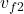=6.5%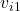Explanation:

Mass of the ball: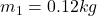]

Initial velocity of the ball:final velocity of the ball: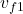which is -30/100 of=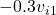Mass of the bottle: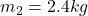Initial velocity of the bottle: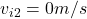final velocity of the bottle:is unknown (to find)

by using conservation momentum, which stated that the initial momentum is equal to the final momentum.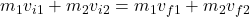so since the bottle is at rest firstly, therefore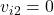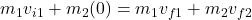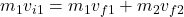equation 1

so now substituteinto equation 1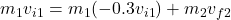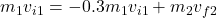collect the like terms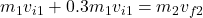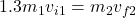divide both  side by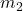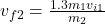Now substitute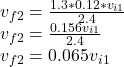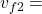6.5%v2 = 6.5% of the ball’s initial velocity

Explanation:

Let the mass of the ball and bottle be m1 and m2 respectively.

m1 = 0.12kg , m2 = 2.4kg

Let the initial and final velocity of ball be u1 and

v1 = -0.3u1 while that of the bottle be u2 and v2

The bottle was initially at rest so u2 = 0m/s

From the principle of conservation of momentum, momentum before collision is equal to momentum after collision.

m1u1 + m2u2 = m1v1 + m2v2

0.12u1 + m2×0 = 0.12(–0.3u1) + 2.4v2

0.12u1 = –0.036u1 + 2.4v2

Collecting like terms

2.4v2 = 0.12u1 + 0.036u1

2.4v2 = 0.156u1

v2 = 0.156u1/2.4

v2 = 0.065u1 = 6.5%u1